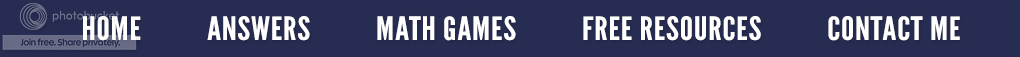### Is Zero Even or Odd?

Is the number zero even or odd?  This was a question asked on the Forum page of Teachers Pay Teachers by an elementary teacher. She stated that Wikipedia had a long page about the parity of zero and that some of the explanation went a little over her head, but basically the gist was that zero is even because it has the properties of an even number. She further stated that before reading this definition, she probably would have said that zero was neither even nor odd.

Here was my reply. Zero is classified as an even number. An integer n is called *even* if there exists an integer m such that n = 2m,  and *odd* if 2m + 1. From this, it is clear that 0 = (2)(0) is even. The reason for this definition is so that we have the property that every integer is either even or odd.

In a simpler format, an even number is a number that is exactly divisible by 2. That means when you divide by two the remainder is zero. You may want your students to review the multiplication facts for 2 and/or other numbers to look for patterns.

2 x 0 =               3 x 0 =
2 x 1 =               3 x 1 =
2 x 2 =               3 x 2 =
2 x 3 =               3 x 3 =

There is always a pattern of the products. Let the students discover these patterns - Even x Even = Even, Even x Odd = Even and vice versa and Odd x Odd = Odd. Since ALL math is based on patterns, seeing patterns in math helps students to understand and remember. Now ask yourself, "Does zero fit this pattern?"

The students can also divide several numbers by 2 (including 0), allowing them to see a second way to conclude that a number is even. (The remainder of the evens is 0, and the remainder of the odds is 1).  Again, "Does zero fit this pattern?"

To demonstrate odds and evens, I like using my hands and fingers since they are always with me. Let's begin with the number two.  I start by having the students make two fists that touch each other. I then have them put one finger up on one hand and one finger up on the other hand. Then the fingers are to make pairs and touch each other. If there are no fingers left over (without a partner), then the number is even.  (see sequence below)

Let's try the same procedure using the number three. Again, begin with the two fists. (see sequence below) Alternating the hands, have the students put up one finger on one hand and one finger up on the other hand; then another finger up on the second hand.  Now have the students make pairs of fingers. Oops!  One of the fingers doesn't have a partner, (one is left over); so, the number three is odd. (I like to say, "Odd man out.")

So, does this work for zero?  If we start with two fists, and put up no fingers then there are no fingers left over.  The fists are the same, making zero even. (see illustration below)

So the next time you are working on odd and even numbers, make it a "hands-on" activity.